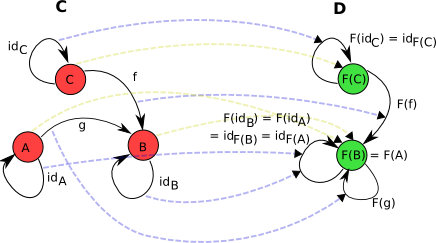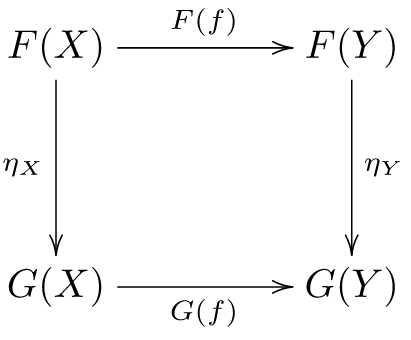# Category

• 一些 对象(object)构成的类 $ob(C)$
• 范畴 $C$ 中对象之间的映射，称为 态射(morphism)。对于范畴 $C$ 中任意两个object $A$、$B$，所有的 $A \to B$ 态射的集合用 $hom(A, B)$ 表示，其中 $A$ 称为domain，$B$称为codomain
• 态射的复合运算($\circ$)，用于将多个态射进行复合。比如 $f: \ A \to B$, $g: \ B \to C$，则$g \circ f: \ A \to C$

$$id(B) \circ f = f = f \circ id(A)$$

• object对应着某个具体的函数（类型？）（这是我的理解，也有很多人认为对象对应着某一类值的集合，即类型。我的理解是type和type constructor都可以看作是function
• 态射对应着函数之间的映射，这种映射产生新的函数。比如 $f :: (a \to b) \to a \to b$
• 态射的复合运算代表着函数的复合（复合函数），比如 h = f . g

# Functor

• 将范畴C的object($X$)映射到范畴D的object ($F(X)$)
• 将范畴C的morphism($f: \ X \to Y$)映射到范畴D的morphism($F(f) : \ F(x) \to F(Y)$)

• 单位态射关系：$\forall X \in C, F(id_{X}) = id_{F(X)}$
• 分配率：$\forall f:X \to Y, g: Y \to Z \in C, F(g \circ f) = F(g) \circ F(f)$$$(a \to b) \to f \ a \to f \ b$$

# Bifunctor

Bifunctor(二元函子)与普通的Functor区别之处在于Bifunctor的domain为两个范畴的积，比如 $F: \ C_{1} \times C_{2} \to D$。

# Hom-functor

• covariant hom-functor: $Hom(A, -): \ C \to Set$，通常称为 copresheaf
• contravariant hom-functor: $Hom(-, A): \ C^{OP} \to Set$，通常称为 presheaf

# Representable functor

$$Hom_{C}(F(-), -) \cong Hom_{D}(-, G(-))$$

$$Hom_{C}(F(Y), X) \cong Hom_{D}(Y, G(X))$$

• 常见的一对伴随函子：Forgetful Functor/Free Functor。$Forget : \ C \to Set$，$Free : \ Set \to C$，$Free \dashv Forget$。

# Natural Transformation• $T$ 为范畴 $X$ 上的自函子： $T: X \to X$
• $\eta$ 为单位自函子 $id_{X}$ 到函子 $T$ 的自然变换：$\eta: id_{X} \to T$
• $\mu$ 为函子 $T$ 的张量积 $T \circ T$ 到函子 $T$ 的自然变换：$\mu: T \circ T \to T$

• $\mu \circ T \mu = \mu \circ \mu T$ (即自然变换 $T^{3} \to T$)
• $\mu \circ T \eta = \mu \circ \eta T = 1_{T}$ (即自然变换 $T \to T$)# TODO

• Monoidal Category / Monoidal Functor